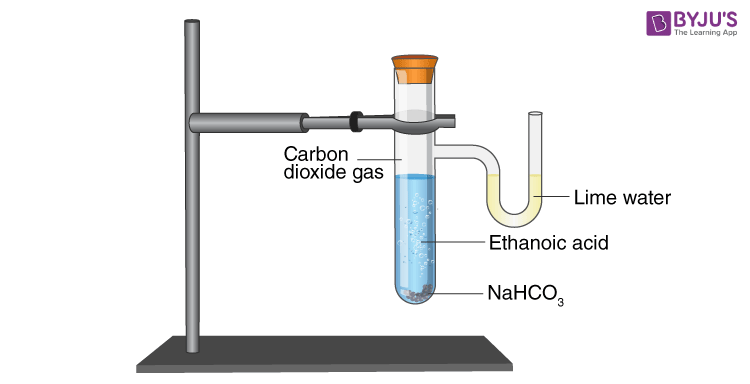Question

# A salt X is formed and a gas is evolved when ethanoic acid reacts with sodium hydrogen carbonate. Name the salt X and the gas evolved. Describe an activity and draw the diagram of the apparatus to prove that the evolved gas is the one which you have named. Also, write a chemical equation of the reaction involved.

Open in App
Solution

## Chemical reactionChemical reactions, in which reactants combine to form products, are represented by chemical equations.Stoichiometric coefficients are added to the reactants and products to balance chemical equations.It's significant because the principles of mass conservation and constant proportions require a chemical equation to be applied.Ethanoic acid reacts with sodium hydrogen carbonateWhen ethanoic acid reacts $\left({\mathrm{CH}}_{3}\mathrm{COOH}\right)$ with sodium hydrogen carbonate $\left({\mathrm{NaHCO}}_{3}\right)$, a salt X is formed and gas is produced.The reaction is as follows:${\mathrm{CH}}_{3}\mathrm{COOH}+{\mathrm{NaHCO}}_{3}\to {\mathrm{CH}}_{3}\mathrm{COONa}+{\mathrm{H}}_{2}\mathrm{O}+{\mathrm{CO}}_{2}$When sodium hydrogen carbonate and ethanoic acid react, sodium ethanoate $\left({\mathrm{CH}}_{3}\mathrm{COONa}\right)$ and carbon dioxide $\left({\mathrm{CO}}_{2}\right)$ gas are produced.Hence, X salt is Sodium ethanoate and gas is carbon dioxide.ActivityIn a test tube, add $2\mathrm{mL}$ of diluted ethanoic acid and a spoonful of sodium hydrogen carbonate.In a test tube, brisk effervescence occurs.Pass the produced gas into lime water.The evolution of carbon dioxide is confirmed when lime water turns milky.Diagram of the apparatusSuggest Corrections1Similar questionsExplore more